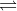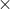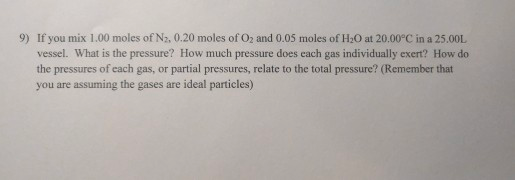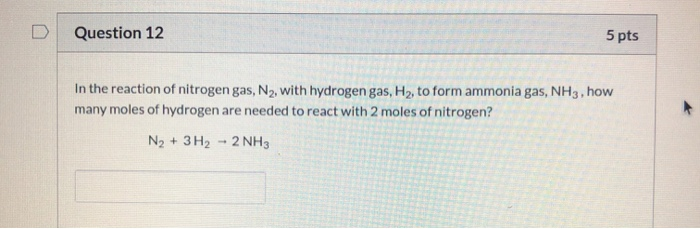Question

# If you mix 2.00 moles of H2, 1.00 moles of N2 and 2.00 moles of NH3...

If you mix 2.00 moles of H2, 1.00 moles of N2 and 2.00 moles of NH3 in a one liter vessel at 472 C. Will H2 and N2 react to form NH3?

As we know that according to the Haber's process of ammonia (NH3), ammonia (NH3) is formed as follows:-

N2(g)   + 3H2(g)2NH3(g)

1 mol 3 mol 2 mol

suppose above mixture present in one liter vessel then

molar concentration of N2 i.e [ N2 ] = no. of moles of N2 / volume of vessel = 1 / 1 = 1 M

molar concentration of H2 i.e [ H2 ] = no. of moles of H2 / volume of vessel = 3 / 1 = 3 M

molar concentration of NH3 i.e [ NH3 ] = no. of moles of NH3 / volume of vessel = 2 / 1 = 2 M

Equilibrium constant of reaction as follows:-

Equilibrium constant (Keq) = [ NH3 ]2 / [ N2 ][ H2 ]3

Equilibrium constant (Keq) = [ 2]2 / [ 1][ 3]3

Equilibrium constant (Keq) = 22 /1333

Equilibrium constant (Keq) = 4 /27

Equilibrium constant (Keq) = 0.148

Given:-

volume of vessel = 1 liter

molar concentration of N2 i.e [ N2 ] = no. of moles of N2 / volume of vessel = 1 / 1 = 1 M

molar concentration of H2 i.e [ H2 ] = no. of moles of H2 / volume of vessel = 2 / 1 = 2 M

molar concentration of NH3 i.e [ NH3 ] = no. of moles of NH3 / volume of vessel = 2 / 1 = 2 M

as we know that

N2(g)   + 3H2(g)2NH3(g)

1 mol 2 mol 2 mol

then  Equilibrium constant of reaction as follows:-

Equilibrium constant (K'eq) = [ NH3 ]2 / [ N2 ][ H2 ]3

Equilibrium constant (K'eq) =   [ 2]2 /[ 1][ 2]3

Equilibrium constant (K'eq) = 22 / 1222

Equilibrium constant (K'eq) = 4 / 8

Equilibrium constant (K'eq) = 0.50

As we know that

Equilibrium constant (K'eq) = [ NH3 ]2 / [ N2 ][ H2 ]3

If the value of Equilibrium constant (Keq) increases then its indicates that the increase the formation of NH3  i.e N2 and

H2 reacts with each other to form NH3 in other words Value of Equilibrium constant (Keq) increase with decrease the concentration of  N2 and H2 and vice versa.

Since the value of Equilibrium constant (K'eq) of the given reaction i.e K'eq = 0.50 is increased as compared to the value of Equilibrium constant (Keq) of the haber's process of the formation of ammonia i.e Keq = 0.148.

As we know that

-Since the value of equilibrium constant (Keq) is independent of the original concentration of the reactants because it has a definite value for every reaction at a given temperature.

- Value of equilibrium constant (Keq) telles us the extent to which the forward or backward reaction has taken place. greater value of equilibrium constant (Keq) means that the reaction proceeded to a greater extent in the forword direction i.e the formation of product (NH3 ).

- As we know that the formation of ammonia (NH3 ) through haber's process is exothermic reaction therefore decrease the temperature of the reaction which leads the formation of ammonia (NH3 ) according to the Le-Chatelier's principle.

The answer is H2 and N2 will react to form NH3 when we mixed 2.00 moles of H2, 1.00 moles of N2 and 2.00 moles of NH3 in a one liter vessel at 472 C.

#### Earn Coins

Coins can be redeemed for fabulous gifts.

Similar Homework Help Questions
• ### 3 H2 + N2 → 2 NH3 1. If you have 3.22 moles of H2, how...

3 H2 + N2 → 2 NH3 1. If you have 3.22 moles of H2, how many moles of NH3 can you make? 2. If you have 1.19 moles of H2, how many moles of NH3 can you make? 3. If you have 15.22 moles of N2, how many moles of NH3 can you make? 4. How many moles of H2 do you need to react with 5.55 moles of N2? 5. How many moles of NH3 can be made...

• ### 9) If you mix 1.00 moles of N2, 0.20 moles of O2 and 0.05 moles of...9) If you mix 1.00 moles of N2, 0.20 moles of O2 and 0.05 moles of H2O at 20.00°C in a 25.00L vessel. What is the pressure? How much pressure does each gas individually exert? How do the pressures of each gas, or partial pressures, relate to the total pressure? (Remember that you are assuming the gases are ideal particles)

• ### 89. A 1.00-L vessel at 400 °C contains the following equilibrium concentrations: N2, 1.00 M; H2,...

89. A 1.00-L vessel at 400 °C contains the following equilibrium concentrations: N2, 1.00 M; H2, 0.50 M; and NH3, 0.25 M. How many moles of hydrogen must be removed from the vessel to increase the concentration of nitrogen to 1.1 M? The equilibrium reaction is N2(g) + 3H2(g) ⇌ 2NH3(g)

• ### Diatomic N2 can react with diatomic H2 to form ammonia (NH3). The balanced chemical equation is:  ...

Diatomic N2 can react with diatomic H2 to form ammonia (NH3). The balanced chemical equation is:   N2 + 3H2 ® 2NH3 If 3 moles of N2 totally reacted with more than enough H2, how many moles of ammonia would be expected to form? 6 moles 4 moles 3 moles 2 moles

• ### 1.00 mol of N2 and 3.00 moles of H2 are mixed together to produce ammonia according...

1.00 mol of N2 and 3.00 moles of H2 are mixed together to produce ammonia according to the equation below. At equilibrium in a 1.00 L vessel, only 30.0% of the N2 that was present originally, remains. Calculate Kc for this reaction at this temperature.

• ### 2.00 g H2 is allowed to react with 9.82 g N2, producing 2.92 g NH3. What...

2.00 g H2 is allowed to react with 9.82 g N2, producing 2.92 g NH3. What is the theoretical yield in grams for this reaction under the given conditions? What is the percent yield for this reaction under the given conditions?

• ### 3H2(g)+N2(g)→2NH3(g) a. How many moles of NH3 can be produced from 13.5 mol of H2 and...

3H2(g)+N2(g)→2NH3(g) a. How many moles of NH3 can be produced from 13.5 mol of H2 and excess N2? b. How many grams of NH3 can be produced from 4.90 mol of N2 and excess H2. c. How many grams of H2 are needed to produce 12.74 g of NH3? d. How many molecules (not moles) of NH3 are produced from 1.05×10−4 g of H2?

• ### If one starts with 50g of N2, how many grams of H2 are required to react...

If one starts with 50g of N2, how many grams of H2 are required to react with the 50 grams of N2? How many moles of NH3 are produced? If one begins with 30g of H2, how many grams of N2 are required? How many grams of NH3 are produced? How many moles of NH3 is that? Please show work !

• ### Question 12 5 pts In the reaction of nitrogen gas, N2, with hydrogen gas, H2, to...Question 12 5 pts In the reaction of nitrogen gas, N2, with hydrogen gas, H2, to form ammonia gas, NH3, how many moles of hydrogen are needed to react with 2 moles of nitrogen? N2 + 3H2 - 2 NH3

• ### A reaction vessel contains NH3, N2, and H2 at equilibrium at a certain temperature. The equilibrium...

A reaction vessel contains NH3, N2, and H2 at equilibrium at a certain temperature. The equilibrium concentrations are [N2] = 0.61 M, [H2] = 1.26 M, and [NH3] = 0.30 M. Calculate the equilibrium constant, Kc, if the reaction is represented as ( 1 2 ) N2(g) + ( 3 2 ) H2(g) ⇌ NH3(g)